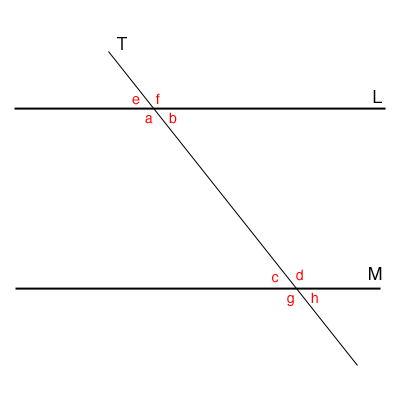SEARCH HOMEMath Central Quandaries & QueriesQuestion from Jillian, a student: I don't understand finding angels in a transversalHi Jillian,

A transversal is a line that crosses two other lines. In many situations the two lines it crosses are parallel. I drew a diagram where the two lines $L$ and $M$ are parallel and the transversal is called $T.$There are eight angles and I have labeled their measures by $a, b, \cdots , h.$ When two lines cross the opposite angles have the same measure so $a = f, b = e, c = h \mbox{ and } d = g.$

If the lines $L$ and $M$ are parallel then $a = d \mbox { and } c = b.$ if $L$ and $M$ are not parallel then $a \ne d \mbox { and } c \ne b.$

There is a nice interactive diagram on the Math Open Reference site.

I hope this helps,
PennyMath Central is supported by the University of Regina and The Pacific Institute for the Mathematical Sciences.